# Designing the Feedback Voltage Resistor Divider in a DC/DC ConverterGet valuable resources straight to your inbox - sent out once per month

## Introduction

The specifications of the feedback (FB) voltage resistor divider in DC-DC converters often poses various design challenges, such as determining the required resistance or adjusting parameters (e.g. output voltage, upper divider resistance or lower divider resistance). Figure 1 shows the various magnitude combinations for the FB upper and lower divider resistances.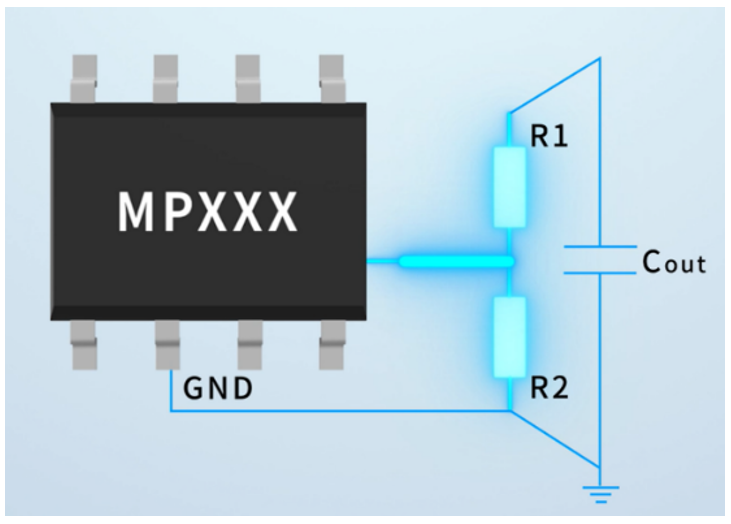Figure 1: Various Magnitude Combinations for FB Upper and Lower Divider Resistances

This article examines the design specifications of the FB voltage resistor divider, including standby power consumption, output voltage accuracy, and loop characteristics.

## Standby Power Consumption

Figure 2 shows the different orders of magnitudes between the DC/DC converter and FB voltage resistor divider with a low quiescent current (IQ). Consider the MPQ4430 where R1 and R2 are the divider resistors.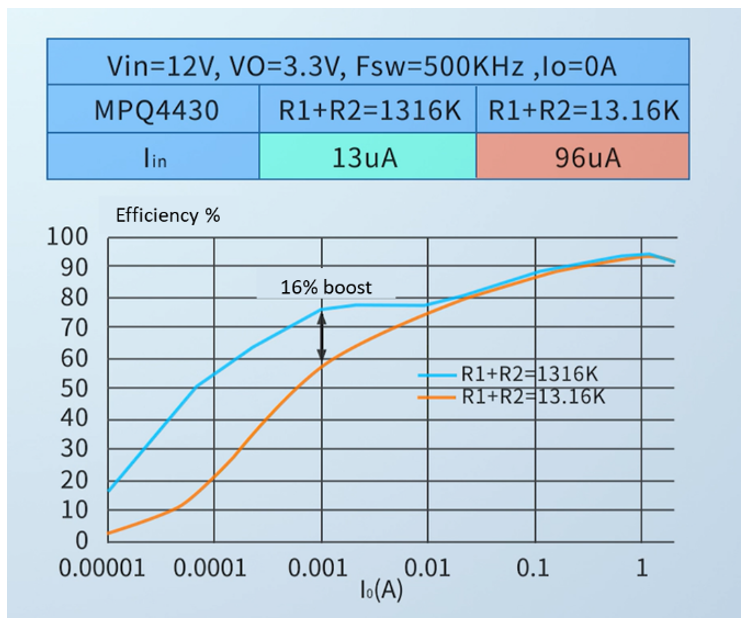Figure 2: Efficiency Difference Caused by Different Orders of R1 and R2 Magnitudes

Optimize the standby power consumption, especially with products that use batteries, to proportionally increase the DC/DC converter’s FB voltage resistor divider value.

## Output Voltage Accuracy

To reduce standby power consumption, it is necessary to increase the resistance of the FB voltage resistor divider.

FB is the operational amplifier’s (op amp) negative input terminal that draws a set current. When the proportion of the FB current (IFB) in the voltage resistor divider network is relatively small, IFB can be ignored. When the proportion is large, meaning the voltage resistor divider’s selected value is large, IFB cannot be disregarded (see Figure 3).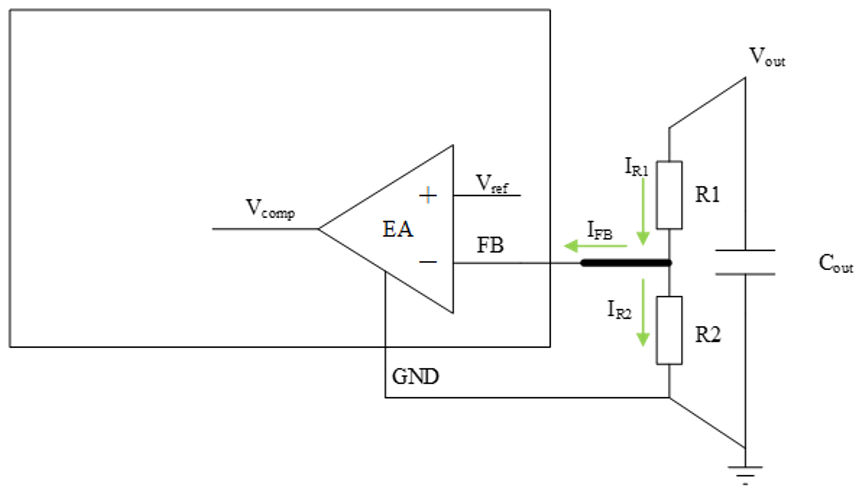Figure 3: Proportion of FB Current in the Voltage Resistor Divider Network

Figure 4 shows that when the actual output voltage (VOUT) exceeds the preset value due to the presence of IFB, poor voltage accuracy results.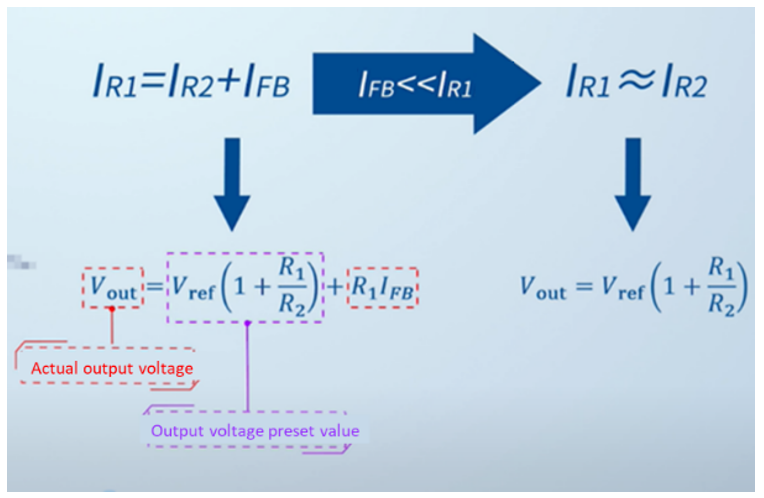Figure 4: Calculated Output Voltage Accuracy

Therefore, the current flowing through the voltage resistor divider when selecting the resistance is recommended to exceed IFB x 50.

## Loop Characteristics

The FB resistance also impacts the loop characteristics inside the chip. When the FB network is comprised of a single resistor, the gain of the voltage-mode op amp and the error amplifier (EA) are related to the upper voltage resistor divider (R1). In the scenario where the dynamic load requires ripple, the R1 value can be adjusted for further optimization. Figure 5 shows the voltage-mode op amp.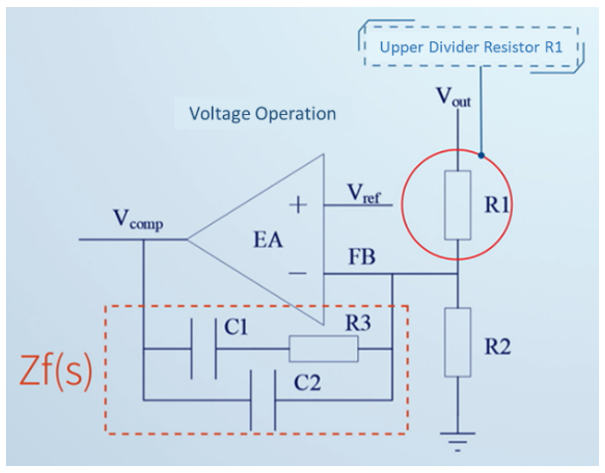Figure 5: Voltage-Mode Operational Amplifier

In the scenario of the current-mode op amp, the op amp gain (GEA(S)) is not directly related to the R1 and R2 values, but is rather related to the ratio of the two resistances. Figure 6 shows the current-mode op amp.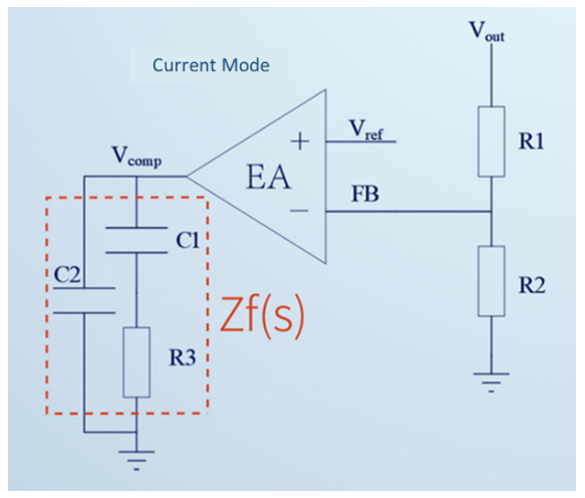Figure 6: Current-Mode Operational Amplifier

Therefore, for different VOUT scenarios (1V, 1.2V, 1.8V, 2.5V, 3.3V, or 5V), it is recommended to keep the resistance of the upper voltage resistor divider constant and change the resistance of the lower voltage resistor divider to obtain the approximate loop characteristics (see Figure 7).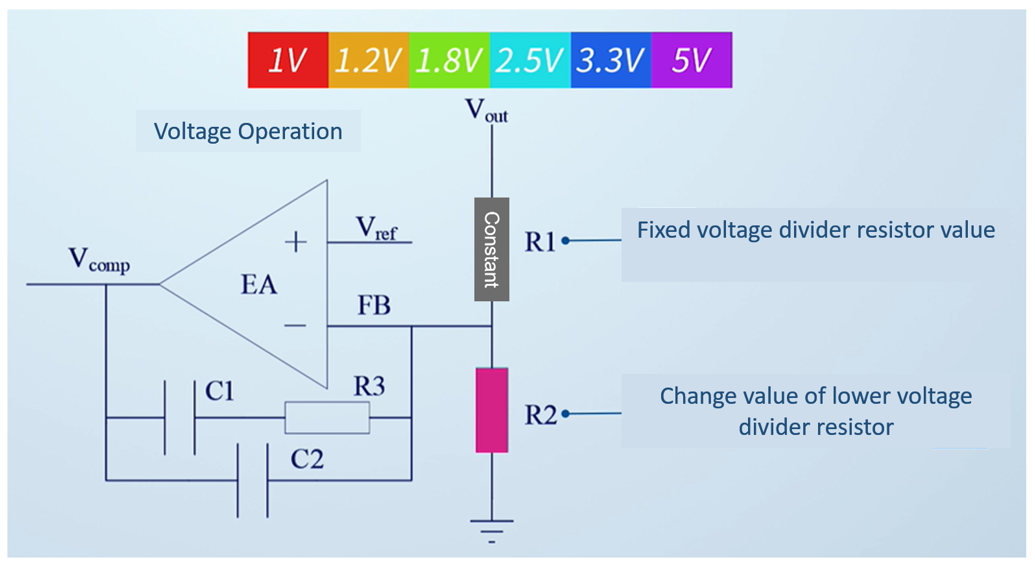Figure 7: Change the Lower Voltage Resistor Divider Resistance with Varying Output Voltages

After obtaining the FB voltage resistor divider value, the FB pin wiring requires attention. FB is a high-impedance pin that can couple noise. In practical applications, R1 and R2 are often placed on the output capacitor’s end, resulting in a long FB trace. This FB trace functions as an antenna and simplifies coupling non-real feedback, which in turn causes VOUT changes or instability (see Figure 8).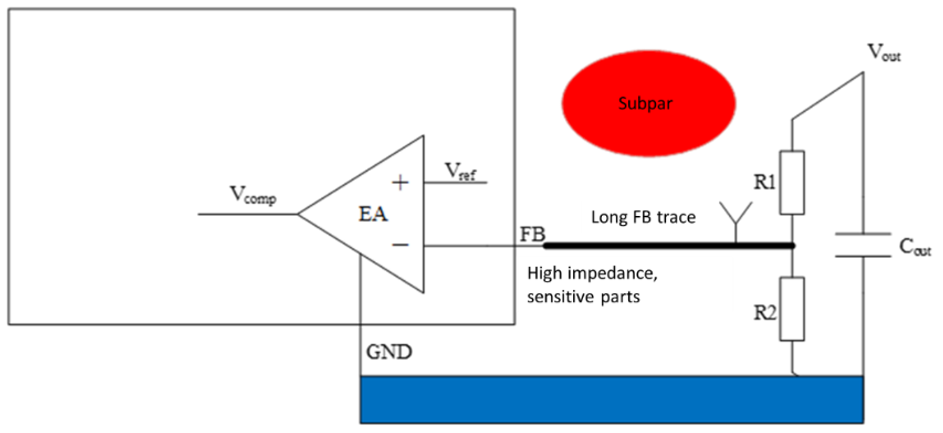Figure 8: Long FB Trace Simplifies Coupling Non-Real Feedback

In the circuit wiring, the FB trace must be as short as possible, R1 and R2 should be placed close to the IC’s FB pin, and VOUT is at DC level with a strong immunity to interference that can be used for long traces (Figure 9).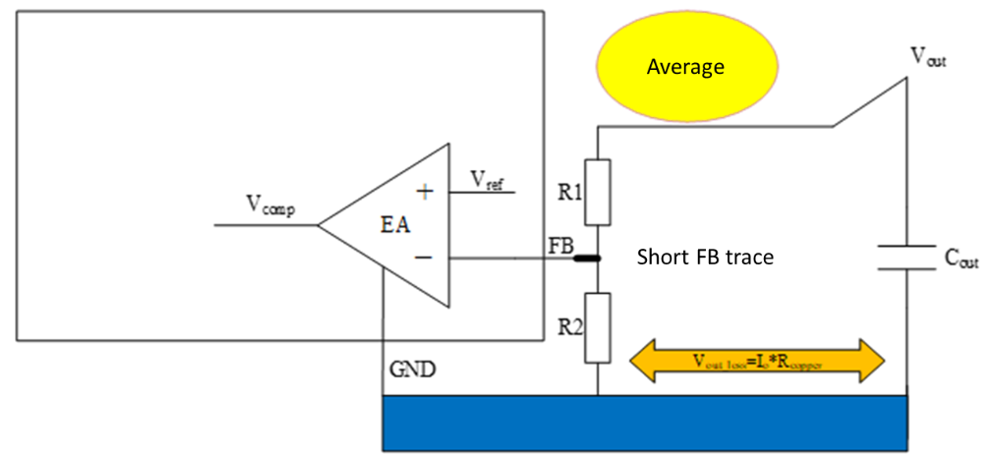Figure 9: Short FB Trace in the Circuit Wiring

Generally, when the output current (IOUT) is only a few amperes, R2’s ground can be connected near the chip ground. However, if IOUT exceeds 10A and the copper layer on the ground trace is limited, the voltage on the ground wire disappears. This results in an actual VOUT below the preset voltage. In this case, remote sampling is recommended (see Figure 10).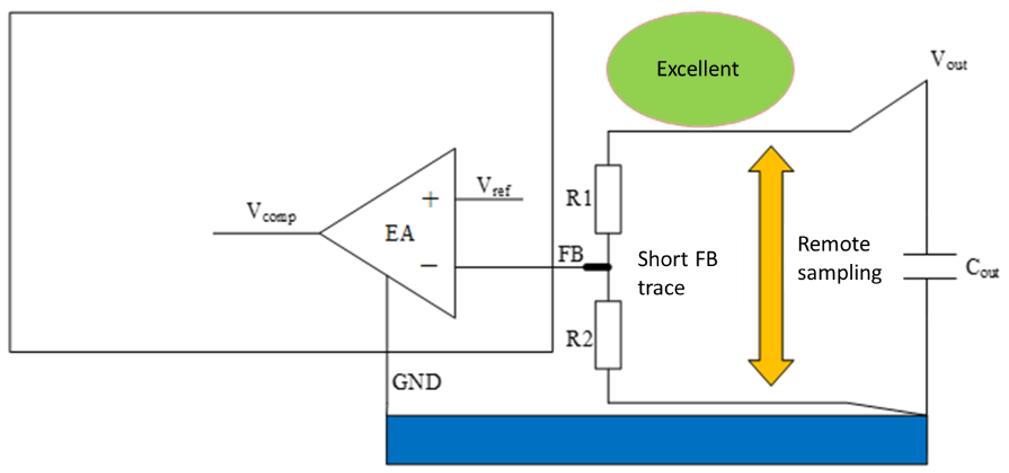Figure 10: Remote Sampling/p>

## Conclusion

To optimize the FB routing in the FB voltage resistor divider in a DC/DC converter, it is important to keep the first element as short as possible and to directly connect the second element on the same side. Ensure that there are no interference sources such as switches, inductors, and an unclean ground.

For more details, explore MPS’s wide selection of switching converters and controllers.

### Did you find this interesting? Get valuable resources straight to your inbox - sent out once per month!

Get technical support